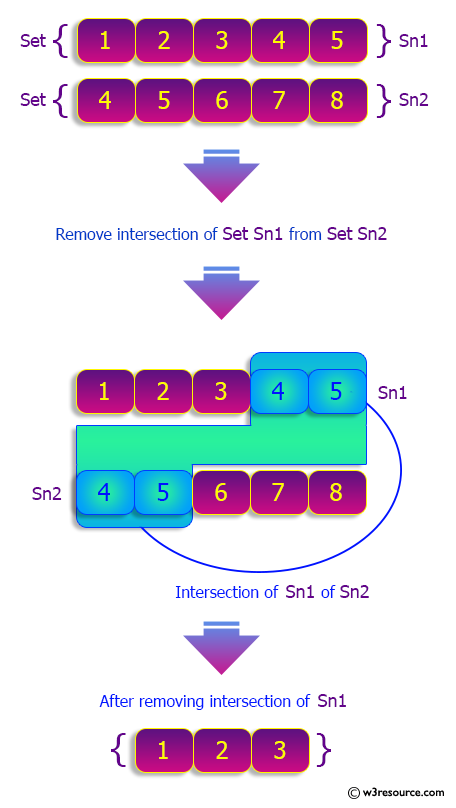﻿ Python: Remove the intersection of a 2nd set from the 1st set - w3resource# Python: Remove the intersection of a 2nd set from the 1st set

## Python sets: Exercise-21 with Solution

Write a Python program to remove the intersection of a 2nd set from the 1st set.

Sample Solution-1:

Python Code:

``````sn1 = {1,2,3,4,5}
sn2 = {4,5,6,7,8}
print("Original sets:")
print(sn1)
print(sn2)
print("\nRemove the intersection of a 2nd set from the 1st set using difference_update():")
sn1.difference_update(sn2)
print("sn1: ",sn1)
print("sn2: ",sn2)
sn1 = {1,2,3,4,5}
sn2 = {4,5,6,7,8}
print("\nRemove the intersection of a 2nd set from the 1st set using -= operator:")
sn1-=sn2
print("sn1: ",sn1)
print("sn2: ",sn2)
```
```

Sample Output:

```Original sets:
{1, 2, 3, 4, 5}
{4, 5, 6, 7, 8}

Remove the intersection of a 2nd set from the 1st set using difference_update():
sn1:  {1, 2, 3}
sn2:  {4, 5, 6, 7, 8}

Remove the intersection of a 2nd set from the 1st set using -= operator:
sn1:  {1, 2, 3}
sn2:  {4, 5, 6, 7, 8}
```

Pictorial Presentation:## Visualize Python code execution:

The following tool visualize what the computer is doing step-by-step as it executes the said program:

Sample Solution-2:

Python Code:

``````sn1 = {1,2,3,4,5}
sn2 = {4,5,6,7,8}
print("Original sets:")
print(sn1)
print(sn2)
print("\nRemove the intersection of a 2nd set from the 1st set using remove():")
for i in sn1&sn2:
sn1.remove(i)

print("sn1: ",sn1)
print("sn2: ",sn2)
```
```

Sample Output:

```Original sets:
{1, 2, 3, 4, 5}
{4, 5, 6, 7, 8}

Remove the intersection of a 2nd set from the 1st set using remove():
sn1:  {1, 2, 3}
sn2:  {4, 5, 6, 7, 8}
```

## Visualize Python code execution:

The following tool visualize what the computer is doing step-by-step as it executes the said program:

Python Code Editor:

Have another way to solve this solution? Contribute your code (and comments) through Disqus.

What is the difficulty level of this exercise?

Test your Python skills with w3resource's quiz

﻿

## Python: Tips of the Day

Trenary operator:

```>>> "Python ROCK" if True else " I AM GRUMPY"
"Python ROCK"
```